Next: Wavefunction of Spin One-Half Up: Spin Angular Momentum Previous: Introduction

# Properties of Spin Angular Momentum

Let us denote the three components of the spin angular momentum of a particle by the Hermitian operators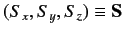. We assume that these operators obey the fundamental commutation relations (297)-(299) for the components of an angular momentum. Thus, we can write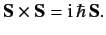(417)

We can also define the operator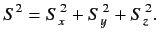(418)

According to the quite general analysis of Section 4.1,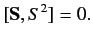(419)

Thus, it is possible to find simultaneous eigenstates of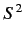and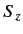. These are denoted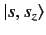, where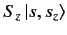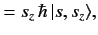(420)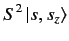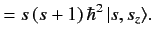(421)

According to the equally general analysis of Section 4.2, the quantum number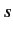can, in principle, take integer or half-integer values, and the quantum number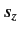can only take the values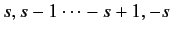.

Spin angular momentum clearly has many properties in common with orbital angular momentum. However, there is one vitally important difference. Spin angular momentum operators cannot be expressed in terms of position and momentum operators, like in Equations (290)-(292), because this identification depends on an analogy with classical mechanics, and the concept of spin is purely quantum mechanical: i.e., it has no analogy in classical physics. Consequently, the restriction that the quantum number of the overall angular momentum must take integer values is lifted for spin angular momentum, since this restriction (found in Sections 4.3 and 4.4) depends on Equations (290)-(292). In other words, the spin quantum numberis allowed to take half-integer values.

Consider a spin one-half particle, for which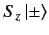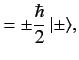(422)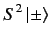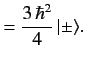(423)

Here, the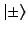denote eigenkets of theoperator corresponding to the eigenvalues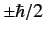. These kets are mutually orthogonal (sinceis an Hermitian operator), so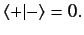(424)

They are also properly normalized and complete, so that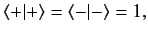(425)

and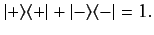(426)

It is easily verified that the Hermitian operators defined by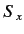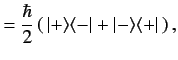(427)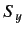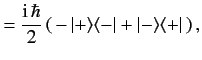(428)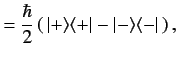(429)

satisfy the commutation relations (297)-(299) (with the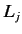replaced by the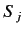). The operatortakes the form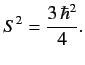(430)

It is also easily demonstrated thatand, defined in this manner, satisfy the eigenvalue relations (422)-(423). Equations (427)-(430) constitute a realization of the spin operators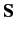and(for a spin one-half particle) in spin space (i.e., the Hilbert sub-space consisting of kets which correspond to the different spin states of the particle).Next: Wavefunction of Spin One-Half Up: Spin Angular Momentum Previous: Introduction
Richard Fitzpatrick 2013-04-08